EMHD Laminar Flow of Bingham Fluid Between Two Parallel Riga Plates

EMHD Laminar Flow of Bingham Fluid Between Two Parallel Riga Plates

Md. Tusher Mollah

Department of Mathematics, European University of Bangladesh, Dhaka 1216, Bangladesh

Corresponding Author Email:
tusher.bsmrstu@gmail.com
Page:
641-648
|
DOI:
https://doi.org/10.18280/ijht.370236
1 April 2019
|
Accepted:
10 June 2019
|
Published:
30 June 2019
| Citation

OPEN ACCESS

Abstract:

The numerical study of EMHD laminar flow of Bingham fluid flowing among two Riga plates with thermal radiation has been established. Both the Riga plates, upper and lower, are taken as stationary. Constant heat transfer is considered for both Riga plates. The governing equations for the problem have been transformed into dimensionless non-linear PDEs by using usual transformations. The obtained non-dimensional PDEs have been solved numerically by applying the explicit FDM. MATLAB has been conducted for the numerical simulation. The stability analysis stated that the governing equations will be converged for $P_{r} \geq 0.09, R_{D} \leq 1.00$ with $\Delta Y=0.05, \Delta \tau=0.0001$. As an outcome, the behavior of several interesting parameters on the flow pattern and on the local shear stress including local Nusselt number have been shown graphically and tabular form. The appropriate mesh space and steady-state solution have been found at $(m, n)=(40,40)$ and $\tau$=4.00 respectively. Finally, a comparison with several published results has been discussed.

Keywords:

MHD flow, Bingham fluid, Riga plate, finite difference scheme, heat transfer

1. Introduction

Bingham fluid is a non-Newtonian viscous fluid that maintains a yield strength which must be surpassed prior to the fluid will flow. It is titled after E.C. Bingham who introduced its mathematical model in 1916 . A most common example is a toothpaste which won't be ejected until certain stress is performed to the tube. Bingham fluid is exploited in several geological and mechanical materials in Mechanical Engineering, Aerospace Engineering as well as Chemical Engineering. Some examples are mud, slurries, cement, sludge, grease, chocolate, etc. On the other hand, the Riga plate is termed as an electromagnetic actuator that is formed by a combination of perpetual magnets and a spanwise aligned pattern of alternating electrodes seated on a plane surface. It is numerously used to avoid the boundary layer separation for the radiation of an efficient agent, pressure drag and skin friction of submarines. The Riga plate is first considered by Gallites and Lilausis  to build an imposed magnetic moreover electric fields those consequently produces Lorentz force collateral to the Riga plate, to control the flow of fluid. The novelty of this research is to introduce Riga plate on the Bingham fluid flow. Many of the researchers describe the Bingham fluid flow and flows along with Riga plate in separate ways.

In this regard, the mathematical representation of Bingham fluid, its constitutive equation, flow characteristics, empirical expression and apparent viscosity have been established in Ref. [3-5]. For the Bingham fluid, the existence of Poiseuille flow, laminar convective heat transfer also the explicit computations of the friction factor have been carried out numerically or analytically by several investigators in Ref. [6-10]. The slow spreading of a sheet of Bingham fluid on an inclined plane, Hall impact and heat transfer about unsteady MHD Couette flow, numerical simulation of Taylor Couette flow, Couette–Poiseuille flow, explicit equations for laminar flow including or excluding suction, injection, slip condition through parallel plate or porous parallel plates or in a channel have been reviewed [11-16]. The MHD Couette flow including thermal radiation, laminar flow with suction and Hall current as well as Ion-slip, unsteady boundary layer flows in a porous medium, viscous incompressible flow including Hall current and MHD two-phase Couette flow for the Bingham fluid through parallel plates or moving plates with heat source and viscous dissipation have been numerically studied [17-23]. For Bingham Plastic, the circular heat and solute source within a viscoplastic porous enclosure: the critical source dimension for optimum transfers has been considered by . Such Couette flow under the action of an imposed magnetic field has vast applications in some systems like; MHD power generators, accelerators, polymer technology, crude oil purification, fluid droplets sprays and petroleum industry.

Separately, the EMHD flow over a Riga-plate, squeezing flow along a Riga plate, nanofluidic transport along Riga plate, radiative flow past a moving or stationary Riga plate Williamson nanofluid over a heated Riga plate, third grade nanofluid along a Riga plate, stagnation point flow in the direction of variable thicked Riga plate, also with or without chemical reaction, with or without suction, injection, and slip condition have been investigated analytically or numerically by many investigators in Ref. [25-32].

Along with the above studies, the present study focuses on the numerical study of EMHD laminar flow of Bingham fluid flowing among two Riga plates with thermal radiation and viscous dissipation. The Ohm’s law including Grinberg term for weakly conducting fluid is concerned with the present study in which this term appeared as an exponential function, as described in the following part and Figure 1. The explicit FDM technique has been used to solve the dimensionless non-linear PDEs. The obtained results have been shown graphically.

2. Mathematical Model Derivation

Consider the EMHD flow of non-Newtonian Bingham fluid which is laminar, incompressible and flows between two infinite horizontal Riga plates which are placed at $y=\pm h$ planes and extend from $x=0$ to $\infty$ and from $z=0 to \infty$ . Both the Riga plates, upper and lower, are taken to be stationary and the uniform velocity outside the boundary is $U_{0}$ . Both Riga plates, lower and upper, are taken at two different constant temperatures $T_{1}$ and $\cdot T_{2}$ respectively, where $T_{2}>T_{1}$. In the X-direction, a constant pressure gradient $\frac{d p}{d x}$ is acted on the fluid. Thus the fluid velocity vector is given as: $\tilde{\boldsymbol{q}}=\tilde{u} \boldsymbol{i}+\tilde{v} \boldsymbol{j}$ .

For the Riga plate the volume density of a Lorentz force is $\boldsymbol{F}=\boldsymbol{J} \wedge \boldsymbol{B}$; where, the current density by means of Ohm’s law is defined as: $J=\sigma(E+\widetilde{q} \wedge B)$

Since the Bingham fluid is weakly conducting ($\sigma=10^{6} \mathrm{S} / \mathrm{mor}$ or very small) then the current density $\sigma(\widetilde{\boldsymbol{q}} \wedge B)$ is small. So that the term $\sigma(\widetilde{\boldsymbol{q}} \wedge B)$ in the above equation, can be neglected. Thus, for finding the electro magneto-hydrodynamic (EMHD) flow, the extrinsic magnetic field is used which is the Lorentz force along the X-axis and is represented as: $\boldsymbol{F}=\boldsymbol{J} \wedge \boldsymbol{B} \approx \sigma(\boldsymbol{E} \wedge \boldsymbol{B})$ the density force F=Fex , in accordance with Grinberg term $\left(\frac{F}{\rho}\right)$, averaged along Z-axis, finally expressed as an exponential function of y, can be expressed as: $F=\frac{\pi}{8} J_{0} M_{0} \exp \left(-\frac{\pi}{a} y\right)$.

1.pngFigure 1. Riga plate and schematic configuration

Within the appearance of the above considerations, the equations connected to the EMHD laminar flow of Bingham fluid among two Riga plates with thermal radiation including viscous dissipation, governed by the system of coupled non-linear PDEs under the boundary-layer approximations, which are furnished as follows:

Continuity equation:

$\frac{\partial \tilde{u}}{\partial x}+\frac{\partial \tilde{v}}{\partial y}=0$(1)

Momntum equation:

\begin{aligned} \frac{\partial \tilde{u}}{\partial t}+\tilde{u} \frac{\partial \tilde{u}}{\partial x}+\tilde{v} \frac{\partial \tilde{u}}{\partial y}=&-\frac{1}{\rho} \frac{d p}{d x}+\frac{1}{\rho} \frac{\partial}{\partial y}\left(\tilde{\mu} \frac{\partial \tilde{u}}{\partial y}\right) \\ &+\frac{\pi}{8 \rho} J_{0} M_{0} \exp \left(-\frac{\pi}{a} y\right) \end{aligned} (2)

Energy equation:

$\frac{\partial \tilde{T}}{\partial t}+\tilde{u} \frac{\partial \tilde{T}}{\partial x}+\tilde{v} \frac{\partial \tilde{T}}{\partial y}=\frac{\kappa}{c_{p} \rho}\left(\frac{\partial^{2} \tilde{T}}{\partial y^{2}}\right)+\frac{\tilde{\mu}}{\rho c_{p}}\left(\frac{\partial \tilde{u}}{\partial y}\right)^{2}$

$+\frac{1}{\rho c_{p}} \frac{16 \sigma^{*}}{3 k^{*}} T_{2}^{3} \frac{\partial^{2} \tilde{T}}{\partial y^{2}}$ (3)

where

$\tilde{\mu}=K+\frac{\tau_{0}}{\left(\frac{\partial \tilde{u}}{\partial y}\right)}$ (4)

and the corresponding initial and boundary conditions for the problem are:

$t \leq 0, \quad \tilde{u}=0, \quad \tilde{T}=T_{1}$ . everywhere (5)

$\tilde{u}=0, \quad \tilde{T}=T_{1} \quad$ at $x=0$

$t>0, \quad \tilde{u}=0, \quad \tilde{T}=T_{1} \quad$ at $y=-h$  (6)

$\tilde{u}=0, \quad \tilde{T}=T_{2} \quad$ at $y=h$

It is required to transform the equations (1) to (4) into dimensionless form, as the solution of these equations with the initial conditions and boundary conditions (5) and (6) will be based on the FDM for numerical solution. The dimensionless quantities that have used are given as follows:

$X=\frac{x}{h}, Y=\frac{y}{L}, U=\frac{\tilde{u}}{U_{0}}, V=\frac{\tilde{v}}{V_{0}}, P=\frac{p}{\rho U_{0}^{2}}, \tau=\frac{t U_{0}}{h}, \overline{\mu}=\frac{\tilde{\mu}}{K}$

and $\theta=\frac{\tilde{T}-T_{1}}{T_{2}-T_{2}},$ where, $V_{0}=\frac{v \pi}{a}, h=\frac{U_{0} L^{2}}{v}, L=\frac{a}{\pi}$ (7)

The obtained dimensionless differential equations are presented as follows:

$\frac{\partial U}{\partial X}+\frac{\partial V}{\partial Y}=0$ (8)

$\frac{\partial U}{\partial \tau}+U \frac{\partial U}{\partial X}+V \frac{\partial U}{\partial Y}=-\frac{d P}{d X}+\frac{1}{R_{e}} \frac{\partial}{\partial Y}\left(\overline{\mu} \frac{\partial U}{\partial Y}\right)+Z e^{-Y}$ (9)

$\frac{\partial \theta}{\partial \tau}+U \frac{\partial \theta}{\partial X}+V \frac{\partial \theta}{\partial Y}=\left(\frac{1}{P_{r}}+\frac{4}{3} R_{D}\right) \frac{\partial^{2} \theta}{\partial Y^{2}}+E_{c} \overline{\mu}\left(\frac{\partial U}{\partial Y}\right)^{2}$ (10)

$\overline{\mu}=1+\frac{\tau_{D}}{\left(\frac{\partial U}{\partial Y}\right)}$ (11)

and the dimensionless conditions are mentioned as follows:

$\tau \leq 0, \quad U=0, \quad \theta=0$ everywhere (12)

$U=0, \quad \theta=0 \quad$ at $X=0$

$\tau>0, \quad U=0, \quad \theta=0 \quad$ at $Y=-1$  (13)

$U=0, \quad \theta=1 \quad$ at $Y=1$

The non-dimensional parameters are given as follows:

$R_{e}=\frac{\rho V_{0} L}{K}$ (Reynolds number); $P_{r}=\frac{\rho c_{p} U_{0} L^{2}}{k h}$ (Prandtl number); $Z=\frac{J_{0} M_{0} a^{2}}{8 \pi \rho U_{0} v}$ (Modified Hartmann number); $E_{C}=\frac{U_{0} K h}{\rho c_{p} L^{2}\left(T_{2}-T_{1}\right)}$ (Eckert number); $R_{D}=\frac{4 \sigma^{*} T_{2}^{3}}{k \kappa^{*}}$ (Radiation parameter) and $\tau_{D}=\frac{\tau_{0} h}{K U_{0}}$ (Bingham number or dimensionless yield stress).

3. Shear Stress and Nusselt Number

The shear stress at upper plate has been studied from the velocity profile. The local shear stress in X- direction for upper wall is $\tau_{L} \equiv \mu\left(\frac{\partial U}{\partial Y}\right)_{Y=1}$. Also the Nusselt number at upper plate has been studied from the temperature profile. The local Nusselt number in X- direction for upper wall is $N u_{L} \equiv \frac{\left(\frac{\partial T}{\partial Y}\right)_{Y=1}}{-\left(T_{m}-1\right)} ;$ where $, T_{m}=\frac{\int_{-1}^{1} U \theta d Y}{\int_{-1}^{1} U d Y}$.

4. Numerical Procedure

A set of finite difference approach is required to solve the dimensionless non-linear PDEs (8) to (11) by the explicit FDM subjected to boundary conditions. So that the region interior to the boundary layer is distributed into a grid of lines perpendicular to Y-axis.

Here it is considered that the height of the plate $X_{\mathrm{max}}(=40)$ i.e. X changes from 0 to 40 and regard $Y_{\mathrm{max}}(=2)$ as corresponding to $Y \rightarrow \infty$ i.e. Y changes from 0 to 2. Also m=40 and n=40 mesh spacing are considered in the X and Y directions respectively as shown in Figure 2.

2.png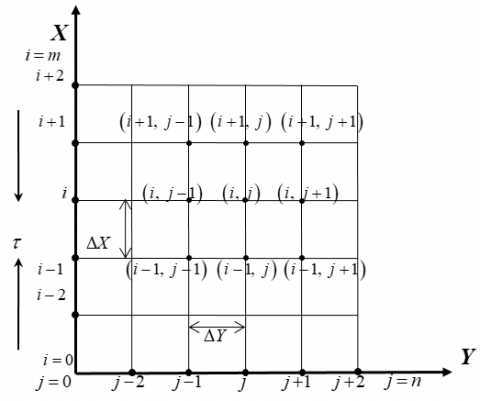Figure 2. Finite difference space grid

It is assumed that $\Delta X$, $\Delta Y$ are constant mesh sizes along X and Y directions respectively and taken as follows:

$\Delta X=1.0(0 \leq x \leq 40), \Delta Y=0.05(0 \leq y \leq 2)$

with the smaller time-step, $\Delta \tau=0.0001$.

Let $U^{\prime}$ and $\theta$ represents the magnitudes of U and θ at the final time-step respectively. With the help of the explicit FDM approach the suitable set of finite difference equations are obtained as follows:

$\frac{U_{i, j}-U_{i-1, j}}{\Delta X}+\frac{V_{i, j}-V_{i, j-1}}{\Delta Y}=0$ (14)

$\frac{U_{i, j}^{\prime}-U_{i, j}}{\Delta \tau}+U_{i, j} \frac{U_{i, j}-U_{i-1, j}}{\Delta X}+V_{i, j} \frac{U_{i, j}-U_{i, j-1}}{\Delta Y}=-\frac{d P}{d X}+Z e^{-Y}+\frac{1}{R_{e}}\left[\begin{array}{c}{\left(\frac{\overline{\mu}_{i, j}-\overline{\mu}_{i, j-1}}{\Delta Y}\right)\left(\frac{U_{i, j}-U_{i, j-1}}{\Delta Y}\right)} \\ {+\overline{\mu}_{i, j}\left(\frac{U_{i, j+1}-2 U_{i, j}+U_{i, j-1}}{(\Delta Y)^{2}}\right)}\end{array}\right]$(15)

$\frac{\theta_{i, j}^{\prime}-\theta_{i, j}}{\Delta \tau}+U_{i, j} \frac{\theta_{i, j}-\theta_{i-1, j}}{\Delta X}+V_{i, j} \frac{\theta_{i, j}-\theta_{i, j-1}}{\Delta Y}=$

$\left(\frac{1}{P_{r}}+\frac{4}{3} R_{D}\right) \frac{\theta_{i, j+1}-2 \theta_{i, j}+\theta_{i, j-1}}{(\Delta Y)^{2}}+E_{c}\left(\overline{\mu}_{i, j}\right)\left(\frac{U_{i, j}-U_{i, j-1}}{\Delta Y}\right)^{2}$ (16)

$\overline{\mu}_{i, j}=1+\frac{\tau_{D}}{\left(\frac{U_{i, j}-U_{i, j-1}}{\Delta Y}\right)}$ (17)

and the boundary conditions with FDM are:

\begin{aligned} U_{i, L} &=0, W_{i, L}=0, \theta_{i, L}=0 \text { at } L=-1 \\ U_{i, L} &=0, W_{i, L}=0, \theta_{i, L}=1 \text { at } L=1 \end{aligned}

5. Stability

Excluding the stability and convergence criteria of the FDM, the analysis will remain incomplete since an explicit procedure is being used. For the considered problem the stability condition finally can be termed as follows:

$\frac{U \Delta \tau}{\Delta X}-\frac{|V| \Delta \tau}{\Delta Y}+\left(\frac{1}{P_{r}}+\frac{4 R_{D}}{3}\right) \frac{2 \Delta \tau}{(\Delta T)^{2}} \leq 1$

Using $\Delta Y=0.05, \Delta \tau=0.0001$ and the initial condition, the above equations gives $P_{r} \geq 0.09$ when $R_{D} \leq 1.00$.

6. Outcomes with Explanation

Due to investigate the physical situation of the developed mathematical model, the steady-state numerical values have been computed for the non-dimensional velocity (U) and temperature (θ) profiles within boundary layer by using FDM with the help of MATLAB R2015 software. The total outcomes can be explained in four parts. At first, the mesh sensitivity has been discussed to obtain the appropriate grid spacing for the numerical calculation. The further calculations have been carried out on applying this output. Secondly, time sensitivity has been explained to obtain the steady-state solution. The further calculations have been carried out on applying the above two outputs. Thirdly, the effect of Reynolds number (Re) and modified Hartmann number (Z) on the velocity (U)  and on the temperature (θ) distributions also on the local shear stress at upper Riga plate L) and local Nusselt number at that plate (NuL) are discussed graphically. Furthermore, for brevity, the effect of other parameters like, Prandtl number (Pr), Eckert number (Ec), Radiation parameter (RD) and Bingham number D) are shown in tabulated form. At last, the present result has been compared with several published results to test the stability of outputs.

6.1 Examine mesh sensitivity

3.png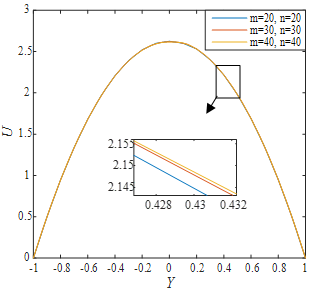Figure 3. Illustration of mesh sensitivity for velocity

To find out the appropriate mesh space i.e. to find the appropriate values of and n, the computations have been continued for three different mesh spaces such as m=20, n=20; m=30, n=30 and m=40, n=40 as shown in Figure 3; where, Re=2.00, Z=0.50, RD=0.05, Ec =0.10, Pr=1.50, and τD=0.001. The obtained curves are smooth and shows a negligible change among these curves. So that any of the above mesh sizes can be chosen as appropriate mesh size. Since it is better to choose the greater mesh size, thus m=40 and n=40 can be chosen as the appropriate mesh size.

6.2 Examine time sensitivity

To complete the time sensitivity test of the developed mathematical model, the computations for U and θ have been continued for different dimensionless time step sizes such as τ=0.50, 1.00, 1.50, 2.00, 2.50, 3.00, 3.50 and 4.00. It is observed that, the result of computations for different profiles, however shows little changes after τ=2.50 and shows negligible changes up to τ= 4.00. Thus the solutions of all variables for τ=4.00 are taken essentially as the steady-state solutions. The time sensitivity for U and θ are shown (see Figure 4).

4a.png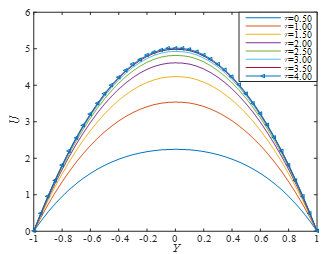(a)

4b.png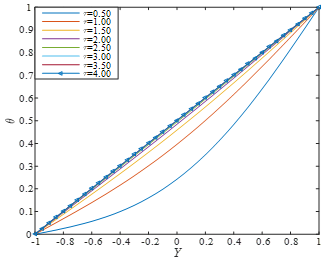(b)

Figure 4. Illustration of time sensitivity for (a) Velocity and (b) Temperature distributions; where, Re=2.00, Z=0.50, RD=0.05, Ec =0.10, Pr=1.50, and τD=0.001

It is seen from Figure 4 that the velocity and the temperature profiles reach their steady state monotonically. It also should be mentioned that the temperature profile reaches the steady state faster than the velocity profile.

6.3 Effect of parameters

In order to get the clear concept of physical phenomena of the developed model, the effects of parameters such as Re and Z, with the appearance of (τD=0.001), (Pr=1.50), (RD=0.001) and (Ec =0.50) are represented graphically through Figures 5-8. For brevity, the impacts of other parameters are presented in Table 1.

The impression of Reynolds number (Re) on U and θ distributions as well as τL and NuL are presented in Figures 5-6. From Figure 5(a, b), it is observed that, the velocity and the temperature distributions increase with the increment of Re. It is seen from Figure 6(a, b) that, number at upper Riga plate, both the local shear stress and Nusselt decreases with the rise of Re.

Furthermore, the effects of modified Hartmann number (Z) on U and θ distributions as well as τL and NuL are presented (see Figures 7-8). From Figure 7(a, b), it is realized that, both the velocity and the temperature distributions increase with the increment of Z . It is seen from Figure 8(a, b) that, at upper Riga plate, both the local shear stress and Nusselt number decreases with the increment of Z .

5a.png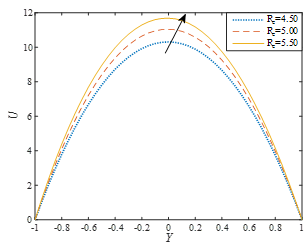(a)

5b.png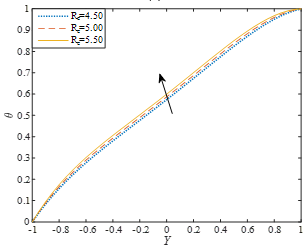(b)

Figure 5. Effects of Re on (a) Velocity and (b) Temperature distributions; where, Z=1.50, RD=0.001, Ec =0.50, Pr=2.50, and τD=0.001 at τ=4.00

Figure 5 shows that, both the distributions increases with the increment of Re.

Figure 6 shows that, at upper Riga plate, both the local shear stress and Nusselt number decreases with the increment of Re.

Figure 7 shows that, both the distributions increase with the increment of Z.

Figure 8 shows that, at upper Riga plate, both the local shear stress and Nusselt number decreases with the rise of Re .

Furthermore, the effects of other parameters like Pr, Ec, RD and τD on θ and NuL are presented in the following tabular form. Table 1 shows that Pr opposes θ and NuL both. Again, Ec enhances θ while it decreases NuL. Furthermore, RD opposes θ while it increases NuL. Also, for τD  a negligible change occurs in θ while τincreases NuL.

6a.png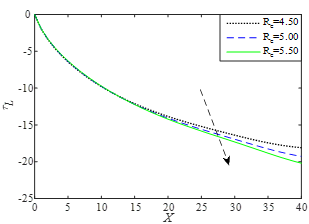(a)

6b.png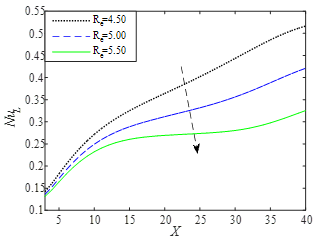(b)

Figure 6. Effects of Re on (a) local shear stress, (b) local Nusselt number at upper Riga plate; where, Z=1.50, RD=0.001, Ec =0.50, Pr=2.50, and τD=0.001 at τ=4.00

7a.png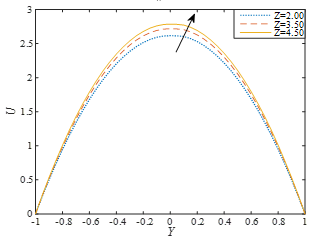(a)

7b.png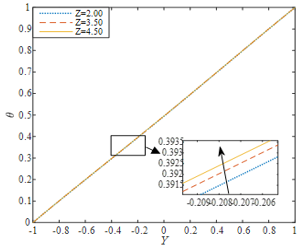(b)

Figure 7. Effects of Z on (a) Velocity and (b) Temperature distributions; where, Re=1.00, RD=0.001, Ec =0.50, Pr=2.50, and τD=0.001 at τ=4.00

8a.png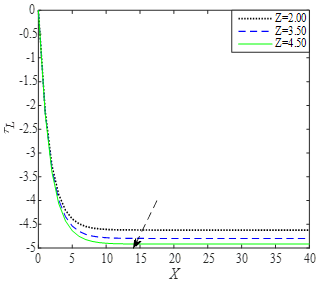(a)

8b.png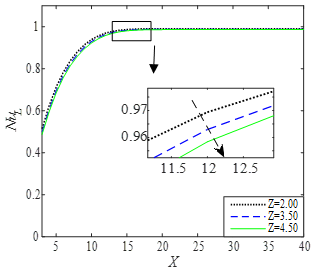(b)

Figure 8. Effects of Z (a) local shear stress, (b) local Nusselt number at upper Riga plate; where, Re=1.00, RD=0.001, Ec =0.50, Pr=2.50, and τD=0.001 at τ=4.00

Table 1. Effects of parameters on temperature distributions & Nusselt number at upper Riga plate

 Effect of Parameters Profiles Pr Ec RD τD θ NuL 1.50 0.50 0.001 0.001 0.5783           0.5781 0.5778 (Dec.) 0.9233           0.9158 0.9081 (Dec.) 1.60 1.70 0.40 0.5774 0.5783 0.5791 (Inc.) 0.9266 0.9233 0.9199 (Dec.) 0.50 0.60 0.001 0.5783          0.5782 0.5764 (Dec.) 0.9233 0.9408 0.9830 (Inc.) 0.100 1.000 0.0001 0.5783 0.5783 0.5783 (Negligible change) 0.9221          0.9227 0.9233 (Inc.) 0.0005 0.0010

6.4 Comparison

A comparison with several published study has presented in the following tabular form. The new invention of the present research is the investigation of the flow of Bingham fluid through Riga plate while Bhatti et al.  studied the viscous nanofluid along a Riga plate with thermal radiation, Ayub et al.  considered nanofluid flow through Riga plate with slip effect, Ahmed et al.  studied the nanofluid flow through Riga plate with Buoyancy effect, Hayat et al.  considered the Squeezing flow through a Riga plate, and Abbas et al.  studied Cassion nanofluid flow through porous Riga plate.

Table 2. Comparison of the present result with several published result

 Output   Effect on Present Result Bhatti et al.  Ayub et al.  Ahmed et al.  Hayat et al.  Abbas et al.  U θ τL   NuL Modified Hartmann number (Z) Inc. Inc. Dec. Dec. Inc. Inc. Inc. Dec. Inc. Inc. Dec.     Inc. Inc.
7. Conclusion

The explicit FDM solution for the EMHD laminar flow of Bingham fluid through a parallel Riga plates with thermal radiation and viscous dissipation has been established. The results were discussed graphically for two important parameters like Re and Z, on the velocity and the temperature distributions, also on the local shear stress and on the local Nusselt number at the upper plate. For brevity, the effect of other parameters such as Pr, Ec, RD and τD are shown in the tabular form. The total results were discussed in the following four parts:

1. Examine Mesh Sensitivity
2. Examine Time Sensitivity
3. Effect of Some Parameters
4. Comparison with Published Results

Finally, the important findings of this investigation are mentioned as follows:

1. The converged solution is found at Pr ≥0.09,RD≤1.00 with ΔY=0.05 and Δτ=0.0001.
2. The appropriate mesh space is found at (m, n) = (40, 40).
3. The steady-state solution is found at τ=4.00.
4. The temperature profile approaches the steady state faster than the velocity profile.
5. The velocity profile enhanced by Re and Z both.
6. The temperature distributions increased by Re, Z and Ec.
7. The temperature distributions reduced by Pand RD both.
8. The Re and Z opposes the local shear stress and Nusselt number both.
9. The Pr  and Ec reduces the Nusselt number while RD and τD enhance.
Acknowledgement

The author would like to acknowledge Prof. Dr. Md. Mahmud Alam, Khulna University, Khulna, Bangladesh, and Mr. Muhammad Minarul Islam, BSMRSTU, Gopalganj, Bangladesh, for their continual support and for their supervision for the project “Unsteady MHD Bingham Fluid Flow through a Parallel Plate with Ion-slip and Hall Current”, since the current research is a wide extension of this project.

Nomenclature
 $\tilde{u}, \tilde{v}$ velocity components along the direction x,y $T_{1}, T_{2}$ temperatures at lower and upper plates $B_{0}$ uniform magnetic field $c_{p}$ speciﬁc heat at the constant pressure $k^{\prime}$ magnetic permeability $U, V$ dimensionless velocity components $N u_{L}$ dimensionless local Nusselt number at upper plate $R_{e}$ Reynolds number $P_{r}$ Prandtl number $E_{C}$ Eckert number $Z$ modified Hartmann number $R_{D}$ Radiation parameter $J_{0}$ applied current density in the electrodes $M_{0}$ magnetization of the permanent magnets $T_{m}$ dimensionless mean fluid temperature $a$ width of magnets and electrodes $k^{*}$ dimensional form is mean absorption coefficient Greek symbols $\sigma^{*}$ Stefan-Boltzmann constant $\mathcal{P}$ density of the ﬂuid $\tau_{D}$ Bingham number or dimensionless yield stress $\sigma$ electric conductivity of the fluid $\mathcal{K}$ thermal conductivity $\tau$ dimensionless time $\theta$ dimensionless temperature $\tilde{\mu}$ viscosity $\tau_{L}$ dimensionless local shear stress at upper plate
References

 Bingham, E.C. (1916). An investigation of the laws of plastic flow. US Bureau of Standards Bulletin, 13: 309-353. https://doi.org/10.6028/bulletin.304

 Gailitis, A., Lielausis, O. (1961). On a possibility to reduce the hydrodynamical resistance of a plate in an electrolyte. Appl. Magnetohydrodyn, 12: 143-146.

 Buckingham, E. (1921). On plastic flow through capillary tubes. ASTM Proceedings 21: 1154-1156.

 Bingham, E.C. (1922). Fluidity and Plasticity. McGraw-Hill, New York.

 Darby, R., Melson, J. (1981). How to predict the friction factor for flow of Bingham plastics. Chemical Engineering 28: 59-61.

 Begis, D., Glowinski, R. (1983). Chapter VII application to the numerical solution of the two-dimensional flow of incompressible viscoplastic fluids. Studies in Mathematics and Its Applications 15: 233-255. https://doi.org/10.1016/S0168-2024(08)70032-8

 Bird, R.B., Dai, G.C., Yarusso, B.J. (1983). The rheology and flow of viscoplastic materials. Reviews in Chemical Engineering, 1(1): 1-70. https://doi.org/10.1515/revce-1983-0102

 Frigaard, I.A., Howison, S.D., Sobey, I.J. (1994). On the stability of poiseuille flow of a Bingham fluid. Journal of Fluid Mechanics, 263: 133-150. https://doi.org/10.1017/S0022112094004052

 Min, T., Yoo, J.Y., Choi, H. (1997). Laminar convective heat transfer of a Bingham plastic in a circular pipe. II. numerical approach––hydro-dynamically developing flow and simultaneously developing flow. International Journal of Heat Mass Transfer, 40(15): 3689-3701. https://doi.org/10.1016/S0017-9310(97)00004-5

 Sablani, S.S., Shayya, W.H., Kacimov, A. (2003). Explicit calculation of the friction factor in pipeline flow of Bingham plastic fluids: a neural network approach. Chemical Engineering Science, ELSEVIER, 58(1): 99-106. https://doi.org/10.1016/S0009-2509(02)00440-2

 Attia, H.A., Sayed-Ahmedm, M.E. (2004). Hall effect on unsteady MHD Couette flow and heat of a Bingham fluid with suction and injection. Applied Mathematical Modeling, 28: 1027–1045. https://doi.org/10.1016/j.apm.2004.03.008

 Liu, K.F., Mei, C.C. (2006). Slow spreading of a sheet of Bingham fluid on an inclined plane. Journal of Fluid Mechanics, 207: 505-529. https://doi.org/10.1017/S0022112089002685

 Tsangaris, S., Nikas, C., Tsangaris, G., Neofytou, P. (2007). Couette flow of a Bingham plastic in a channel with equally porous parallel walls. Journal of Non-Newtonian Fluid Mechanics, 144(1): 42-8.  https://doi.org/10.1016/j.jnnfm.2007.03.004

 Chen, Y.L., Zhu, K.Q. (2008). Couette–Poiseuille flow of Bingham fluids between two porous parallel plates with slip conditions. Journal of Non-Newtonian Fluid Mechanics, 153: 1–11. https://doi.org/10.1016/j.jnnfm.2007.11.004

 Jeng, J., Zhu, K. (2010). Numerical simulation of Taylor Couette flow of Bingham fluids. Journal of Non-Newtonian Fluid Mechanics, 165: 1161-1170. https://doi.org/10.1016/j.jnnfm.2010.05.013

 Swamee, P.K., Aggarwal, N. (2011). Explicit equations for laminar flow of Bingham plastic fluids. Journal of Petroleum Science and Engineering, 76(3-4): 178-184. https://doi.org/10.1016/j.petrol.2011.01.015

 Naik, S.H., Rao, K.R., Murthy, M.V.R. (2014). The effect of hall current on unsteady MHD free convective Couette flow of a Bingham fluid with thermal radiation. International Journal of Engineering and Advanced Technology (IJEAT), 3(6): 1-16.

 Parvin, A., Dola, T.A., Alam, M.M. (2014). Unsteady MHD Bingham fluid flow with hall current and suction. International Journal of Modern Embedded System (IJMES), 2(5): 20-25.

 Hatami, M., Hosseinzadeh, K., Domairry, G., Behnamfar, M.T. (2014). Numerical study of MHD two-phase Couette flow analysis for fluid-particle suspension between moving parallel plates. Journal of the Taiwan Institute of Chemical Engineers, 45(5): 2238-45. https://doi.org/10.1016/j.jtice.2014.05.018

 Parvin, A., Dola, T.A., Alam, M.M. (2015). Unsteady MHD viscous incompressible Bingham fluid flow with hall current. AMSE JOURNALS–2015-Series: Modelling B, 84(1): 38-48.

 Rees, D.A.S., Bassom, A.P. (2015). Unsteady thermal boundary layer flows of a Bingham fluid in a porous medium. International Journal of Heat and Mass Transfer, 82: 460-467. https://doi.org/10.1016/j.ijheatmasstransfer.2014.10.047

 Mollah, M.T., Islam, M.M., Alam, M.M. (2018). Hall and ion-slip effects on unsteady MHD Bingham fluid flow with suction. Modelling, Measurement and Control B, 87(4): 221-229. https://doi.org/10.18280/mmc_b.870402

 Mollah, M.T., Islam, M.M., Alam, M.M. (2018). Couette flow of Bingham fluid with ion-slip and hall current. Lambert Academic Publishing, Riga, Latvia.

 Ragui, K., Boutra, A., Benkahla, Y.K., Bennacer, R. (2018). Circular heat and solute source within a viscoplastic porous enclosure: The critical source dimension for optimum transfers. International Journal of Heat and Technology, 36(2): 761-772. https://doi.org/10.18280/ijht.360243

 Pantokratoras, A., Magyari, E. (2009). EMHD free-convection boundary-layer flow from a Riga-plate. Journal of Engineering Mathematics, 64(3): 303-315. https://doi.org/10.1007/s10665-008-9259-6

 Ahmad, A., Asghar, S., Afzal, S. (2016). Flow of nanofluid past a Riga plate. Journal of Magnetism and Magnetic Materials, 402: 44-48. https://doi.org/10.1016/j.jmmm.2015.11.043

 Hayat, T., Khan, M., Khan, M.I., Alsaedi, A., Ayub, M. (2017). Electromagneto squeezing rotational flow of Carbon (C)-Water (H2O) kerosene oil nanofluid past a Riga plate: A numerical study. PloS One, 12(8): 1-17. https://doi.org/10.1371/journal.pone.0180976

 Iqbal, Z., Mehmood, Z., Azhar, E., Maraj, E.N. (2017). Numerical investigation of nanofluidic transport of gyrotactic microorganisms submerged in water towards Riga plate. Journal of Molecular Liquids, 234: 296-308. https://doi.org/10.1016/j.molliq.2017.03.074

 Ramzan, M., Bilal, M., Chung, J.D. (2017). Radiative Williamson nanofluid flow over a convectively heated Riga plate with chemical reaction-A numerical approach, Chinese Journal of Physics, 55(4): 1663-1673. https://doi.org/10.1016/j.cjph.2017.04.014

 Ramesh, G.K., Gireesha, B.J. (2017). Non-linear radiative flow of nanofluid past a moving/stationary Riga plate. Frontiers in Heat and Mass Transfer (FHMT), 9(1): 1-7. https://doi.org/10.5098/hmt.9.3

 Naseem, A., Shafiq, A., Zhao, L., Farooq, M.U. (2018). Analytical investigation of third grade nanofluidic flow over a Riga plate using Cattaneo-Christov model. Results in Physics, 9: 961-969. https://doi.org/10.1016/j.rinp.2018.01.013

 Anjum, A., Mir, N.A., Farooq, M., Khan, M.I., Hayat, T. (2018). Influence of thermal stratification and slip conditions on stagnation point flow towards variable thicked Riga plate. Results in Physics, 9: 1021-1030. https://doi.org/10.1016/j.rinp.2018.02.069

 Bhatti, M.M., Abbas, T., Rashidi, M.M. (2016). Effects of thermal radiation and electromagnetohydrodynamics on viscous nanofluid through a Riga plate. Multidiscipline Modeling in Materials and Structures, 12(4): 605-18. https://doi.org/10.1108/MMMS-07-2016-0029

 Ayub, M., Abbas, T., Bhatti, M.M. (2016). Inspiration of slip effects on electromagnetohydrodynamics (EMHD) nanofluid flow through a horizontal Riga plate. The European Physical Journal Plus, 131(6): 193. https://doi.org/10.1140/epjp/i2016-16193-4

 Ahmad, R., Mustafa, M., Turkyilmazoglu, M. (2017). Buoyancy effects on nanofluid flow past a convectively heated vertical Riga-plate: A numerical study. International Journal of Heat and Mass Transfer, 111: 827-835. https://doi.org/10.1016/j.ijheatmasstransfer.2017.04.046

 Hayat, T., Khan, M., Imtiaz, M., Alsaedi, A. (2017). Squeezing flow past a Riga plate with chemical reaction and convective conditions. Journal of Molecular Liquids, 225: 569-76. https://doi.org/10.1016/j.molliq.2016.11.089

 Abbas, T., Bhatti, M.M., Ayub, M. (2018). Aiding and opposing of mixed convection Casson nanofluid flow with chemical reactions through a porous Riga plate. Proceedings of the Institution of Mechanical Engineers, Part E: Journal of Process Mechanical Engineering, 232(5): 519-27. https://doi.org/10.1177/0954408917719791